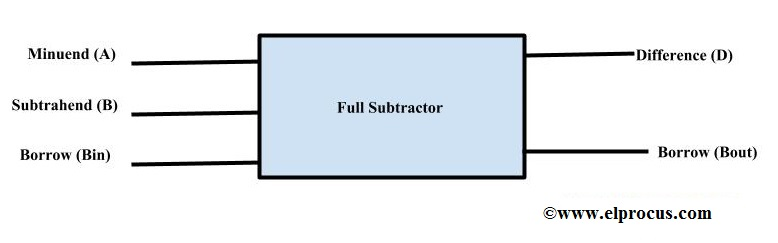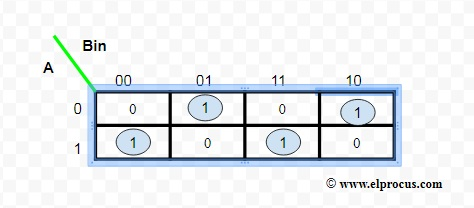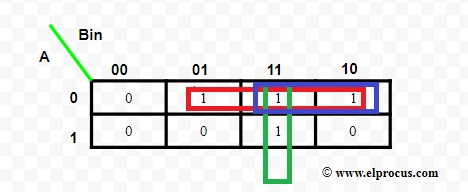# What is a Full Subtractor : Construction using Logic Gates

Generally, the full subtractor is one of the most used and essential combinational logic circuits. It is a basic electronic device, used to perform subtraction of two binary numbers. In the earlier article, already we have given the basic theory of half adder & a full adder which uses the binary digits for the computation. Likewise, the full-subtractor uses binary digits like 0,1 for the subtraction. The circuit of this can be built with logic gates such as OR, Ex-OR, NAND gate. The inputs of this subtractor are A, B, Bin and outputs are D, Bout.

This article gives a full-subtractor theory idea which comprises the premises like what is a subtractor, design with logic gates, truth table, etc. This article is useful for engineering students who can go through these topics in the HDL Practical lab.

## What is a Subtractor?

The binary digits subtraction can be done with the help of the subtractor circuit. This is one kind of combinational logic circuit, used to perform the subtraction of two binary digits like 0s and 1s. Subtraction of binary digits from 0 to 0 or 0 to 1 does not alter the result, subtraction of 1 to 1 will result as 0 but the subtraction of 1 to 0 needs borrow.

For instance, the two-bit subtractor circuit includes two inputs like A & B whereas the outputs are difference and borrow. This circuit can be built with adders along with inverters which are located among every data input as well as borrow (Bin) input of the earlier phase of FA.

Subtractors are classified into two types like half subtractor and full subtractor. Here we are discussing the full subtractor.

### What is a Full Subtractor?

It is an electronic device or logic circuit which performs subtraction of two binary digits. It is a combinational logic circuit used in digital electronics. Many combinational circuits are available in integrated circuit technology namely adders, encoders, decoders, and multiplexers. In this article, we are going to discuss its construction using half subtractor and also the terms like truth table.

The designing of this can be done by two half subtractors, which involves three inputs such as minuend, subtrahend, and borrow, borrow bit among the inputs is obtained from the subtraction of two binary digits and is subtracted from the next higher-order pair of bits, outputs as difference and borrow.

The full subtractor block diagram is shown below. The foremost disadvantage of the half subtractor is, we cannot make a Borrow bit in this subtractor. Whereas in its design, actually we can make a Borrow bit in the circuit & can subtract with the remaining two i/ps. Here A is minuend, B is subtrahend & Bin is borrow in. The outputs are Difference (Diff) & Bout (Borrow out). The complete subtractor circuit can obtain by using two half subtractors with an extra OR gate.### Full Subtractor Circuit Diagram with Logic Gates

The circuit diagram of the full subtractor using basic gates is shown in the following block diagram. This circuit can be done with two half-Subtractor circuits.

In the initial half-Subtractor circuit, the binary inputs are A and B. As we have discussed in the previous half-Subtractor article, it will generate two outputs namely difference (Diff) & Borrow.

The difference o/p of the left subtractor is given to the Left half-Subtractor circuit’s. Diff output is further provided to the input of the right half Subtractor circuit. We offered the Borrow in bit across the other i/p of the next half subtractor circuit. Once more it will give Diff out as well as Borrow out the bit. The final output of this subtractor is Diff-output.

On the other hand, the Borrow out of both the half Subtractor circuits is connected to OR logic gate. Later than giving out OR logic for two output bits of the subtractor, we acquire the final Borrow out of the subtractor. The last Borrow out to signify the MSB (a most significant bit).

If we observe the internal circuit of this, we can see two Half Subtractors with NAND gate and XOR gate with an extra OR gate.

### Full Subtractor Truth Table

This subtractor circuit executes a subtraction between two bits, which has 3- inputs (A, B, and Bin) and two outputs (D and Bout). Here the inputs indicate minuend, subtrahend, & previous borrow, whereas the two outputs are denoted as borrow o/p and difference. The following image shows the truth table of the full-subtractor.

 Inputs Outputs Minuend (A) Subtrahend (B) Borrow (Bin) Difference (D) Borrow (Bout) 0 0 0 0 0 0 0 1 1 1 0 1 0 1 1 0 1 1 0 1 1 0 0 1 0 1 0 1 0 0 1 1 0 0 0 1 1 1 1 1

### K-Map

The simplification of the full subtractor K-map for the above difference and borrow is shown below.The equations for the difference as well as Bin are mentioned below.The expression for Difference is,

D = A’B’Bin + AB’Bin’+ A’BBin’ + ABBin

The expression for Borrow is,

Bout = A’Bin + A’B + BBin

#### Cascading of Full Subtractor Circuit

Previously, we have discussed an overview of this like construction, circuit diagram with logic gates. But if we want to subtract two otherwise more 1-bit numbers, this subtractor circuit is very helpful to cascade single bit numbers and also subtracts more than two binary numbers. In such cases, a full adder cascaded circuit is used with the help of NOT logic gate. The conversion of the circuit from full adder to full subtractor can be done using 2’s complement technique.

Generally, invert the subtrahend inputs for the full adder using NOT gate otherwise an inverter. By adding this Minuend (noninverted input) & Subtrahend (Inverted Input), the LSB (carry input) of the FA circuit is 1, which means Logic High otherwise we subtract two binary digits using 2’s complement technique. The FA’s output is the Diff bit & if we invert the carry out then we can get the MSB otherwise Borrow bit. Actually, we can design the circuit so that output can be observed.

### Verilog Code

For the coding part, first, we need to check the structural way of modeling of the logic circuit diagram. The logic diagram of this can be built using an AND gate, half subtractor circuits, and the combination of logic gates like AND, OR, NOT, XOR gates. As in structural modeling, we explain various modules for every basic elemental arrangement. In the following code, different modules can be defined for every gate.

This module is for the OR gate.

INPUT: a0, b0

OUTPUT: c0

Lastly, we will unite these gate precise modules into an only module. For that, here we utilize instantiation of module. Now this instantiation can be used once we want to replicate an exact module or function for diverse input sets. First, we design a half subtractor then this module is used to implement a full subtractor. For implementing this, we use the OR gate to combine the o/ps for the variable of Bout. The verilog code for the full subtractor is shown below

module or_gate(a0, b0, c0);

input a0, b0;

output c0;

assign c0 = a0 | b0;

endmodule

module xor_gate(a1, b1, c1);

input a1, b1;

output c1;

assign c1 = a1 ^ b1;

endmodule

module and_gate(a2, b2, c2);

input a2, b2;

output c2;

assign c2 = a2 & b2;

endmodule

module not_gate(a3, b3);

input a3;

output b3;

assign b3 = ~ a3;

endmodule

module half_subtractor(a4, b4, c4, d4);

input a4, b4;

output c4, d4;

wire x;

xor_gate u1(a4, b4, c4);

and_gate u2(x, b4, d4);

not_gate u3(a4, x);

endmodule

module full_subtractor(A, B, Bin, D, Bout);

input A, B, Bin;

output D, Bout;

wire p, q, r;

half_subtractor u4(A, B, p, q);

half_subtractor u5(p, Bin, D, r);

or_gate u6(q, r, Bout);

endmodule

#### Full Subtractor using 4X1 Multiplexer

The execution of subtraction can be done through the two’s complement method. Thus we require utilizing a 1-XOR gate which is used to invert 1-bit & include one into carry bit. The output of DIFFERENCE is similar to the output SUM in the full adder circuit however the BARROW o/p is not similar to the full adder’s carry output however it is inverted as well as complimented, like A – B = A + (-B) = A + two’s complement of B.

The design of this using 4X1 multiplexer is shown in the following logic diagram. This design can be done using the following steps.

• In step1, there are two outputs like Sub and Borrow. So we have to choose 2 multiplexers.
• In step2, the truth table can be implemented along with K-maps
• In step3, the two variables can be selected as your select line. For instance, B & C are in this case.

#### Truth Table

The truth table of full subtractor circuit using 4X1 multiplexer includes the following

 A B C Sub Borrow 0 0 0 0 0 0 0 1 1 1 0 1 0 1 1 0 1 1 0 1 1 0 0 1 0 1 0 1 0 0 1 1 0 0 0 1 1 1 1 1

### Full Subtractor using Decoder

The designing of a full subtractor using 3-8 decoders can be done using active low outputs. Let’s assume decoder functioning by using the following logic diagram. The decoder includes three inputs in 3-8 decoders. Based on the truth table, we can write the minterms for the outputs of difference & borrow.

From the above truth table,

For the different functions in the truth table, the minterms can be written as 1,2,4,7, and similarly, for the borrow, the minterms can be written as 1,2,3,7. The 3-8 decoders include three inputs as well as 8 outputs lik0 to 7 numbers.

If the input of the subtractor is 000, then output ‘0’ will be active and if the input is 001, then the output ‘1’ will be active.

Now the outputs of the subtractor can be taken from 1, 2, 4 &7 to connect it to a NAND gate, then the output will be the difference. These outputs can be connected to other NAND logic gates where the output changes to the borrow.

For example, if the input is 001, then the output will be 1 that means it is active. So the output is active low and the output can be obtained from NAND gate called difference function like high and borrow function also changes out to be high. Therefore we get the preferred output. So finally, the decoder works like a full subtractor.

The advantages of the subtractor include the following.

• The designing of subtractor is very simple as well as implement
• Power deduction within DSP (digital signal processing)
• Computational tasks can be carried out at high speed.

The disadvantages of the subtractor include the following.

• In the half subtractor, there is no condition to accept Borrow-like input from the earlier phase.
• The subtractor speed can be partial through the delay in the circuit.

### Applications

Some of the applications of full-subtractor include the following

• These are generally employed for ALU (Arithmetic logic unit) in computers to subtract as CPU & GPU for the applications of graphics to decrease the circuit difficulty.
• Subtractors are mostly used for performing arithmetical functions like subtraction, in electronic calculators as well as digital devices.
• These are also applicable for different microcontrollers for arithmetic subtraction, timers, and the program counter (PC)
• Subtractors are used in processors to compute tables, addresses, etc.
• It is also useful for DSP and networking based systems.
• These are used mainly for ALU within computers for subtracting like CPU & GPU for graphics applications to reduce the complexity of the circuit.
• These are mainly used to perform arithmetical functions such as subtraction within digital devices, calculators, etc.
• These subtractors are also appropriate for various microcontrollers for timers, PC (program counter) & arithmetic subtraction
• These are employed for processors to calculate addresses, tables, etc.
• The implementation of this with logic gates like NAND & NOR can be done with any full subtractor logic circuit because both the NOR & NAND gates are called universal gates.

From the above information, by evaluating the adder, full subtractor using two half subtractor circuits, and its tabular forms, one can notice that Dout in the full-subtractor is accurately similar to the Sout of the full-adder. The only variation is that A (input variable) is complemented in the full-subtractor. Thus, it is achievable to change the full-adder circuit into a full-subtractor by just complementing the i/p A before it is given to the logic gates to generate the last borrow-bit output (Bout).

By using any full subtractor logic circuit, full subtractor using NAND gates and full subtractor using nor gates can be implemented, since both the NAND and NOR gates are treated as universal gates. Here is a question for you, what is the difference between half subtractor and full subtractor?